Скачать презентацию Programming for Geographical Information Analysis Advanced Skills Lecture

47ae34bdf65acd00c0dcfe037446c2d0.ppt

• Количество слайдов: 51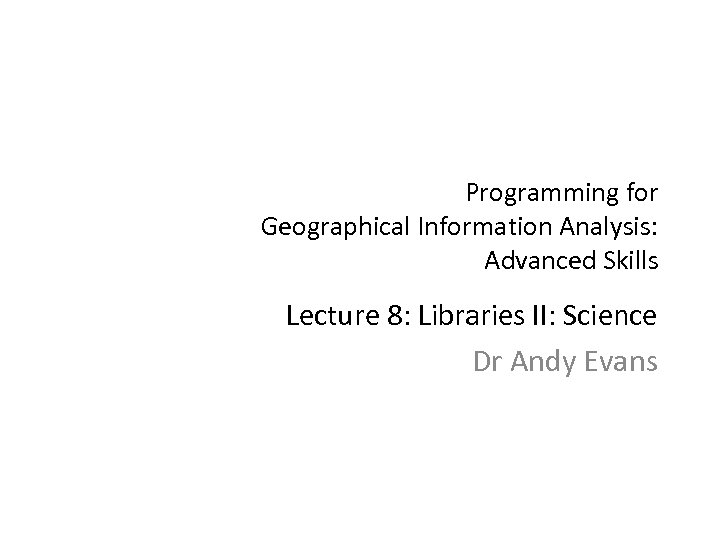Programming for Geographical Information Analysis: Advanced Skills Lecture 8: Libraries II: Science Dr Andy Evans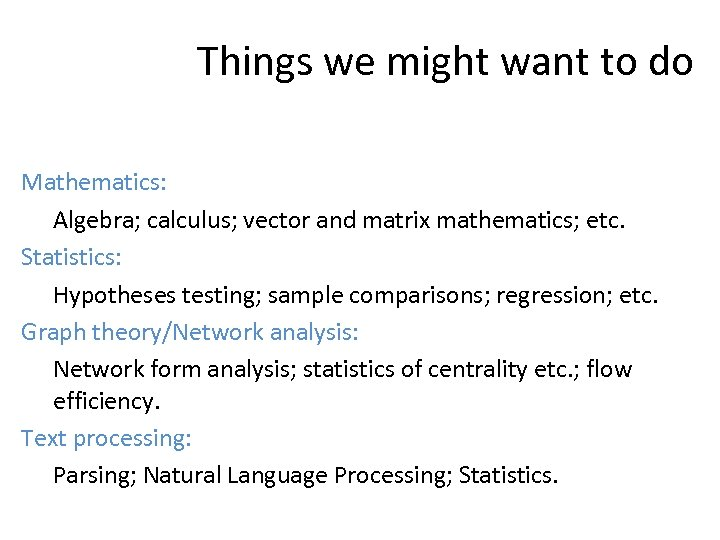Things we might want to do Mathematics: Algebra; calculus; vector and matrix mathematics; etc. Statistics: Hypotheses testing; sample comparisons; regression; etc. Graph theory/Network analysis: Network form analysis; statistics of centrality etc. ; flow efficiency. Text processing: Parsing; Natural Language Processing; Statistics.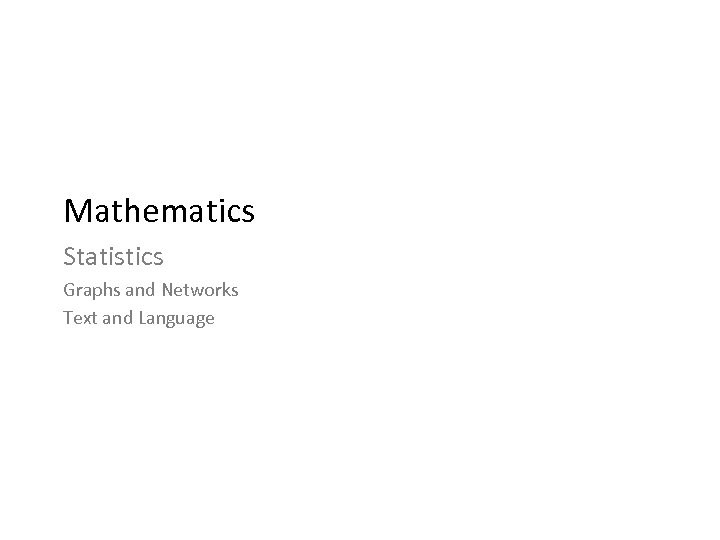Mathematics Statistics Graphs and Networks Text and Language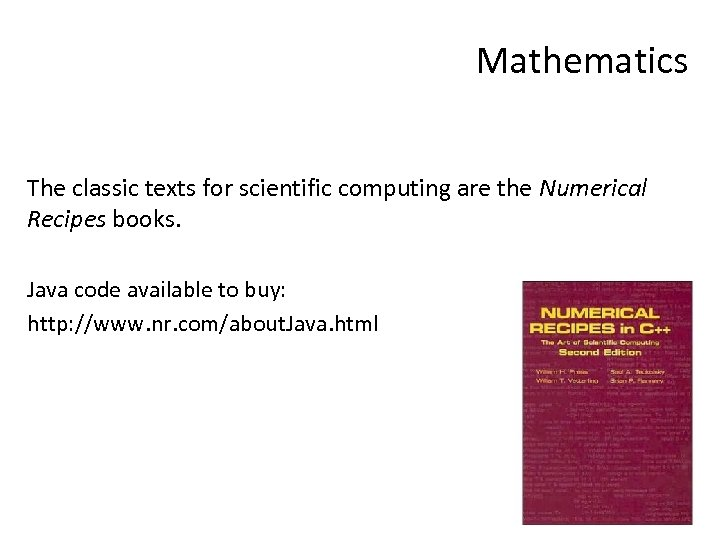Mathematics The classic texts for scientific computing are the Numerical Recipes books. Java code available to buy: http: //www. nr. com/about. Java. html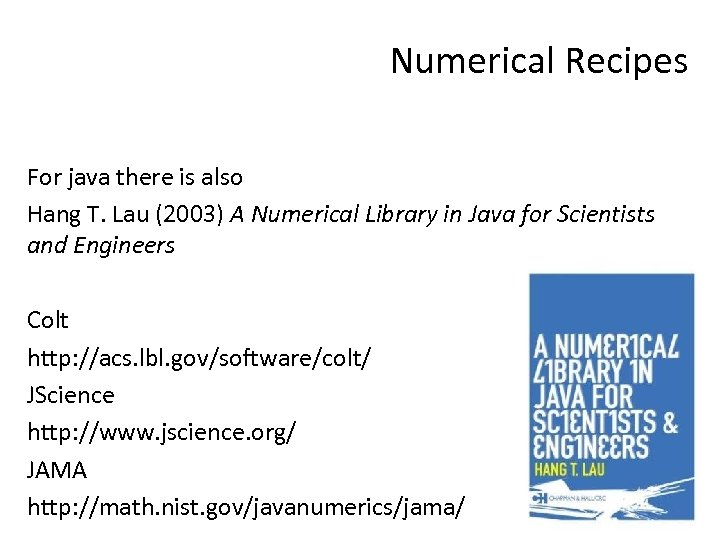Numerical Recipes For java there is also Hang T. Lau (2003) A Numerical Library in Java for Scientists and Engineers Colt http: //acs. lbl. gov/software/colt/ JScience http: //www. jscience. org/ JAMA http: //math. nist. gov/javanumerics/jama/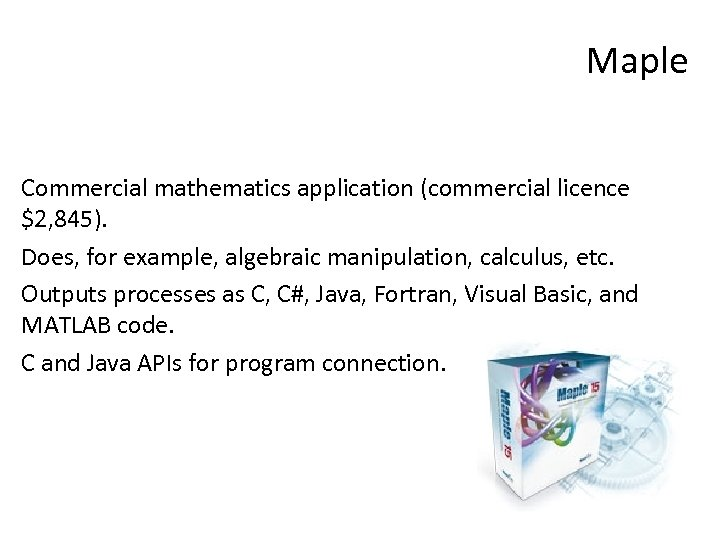Maple Commercial mathematics application (commercial licence \$2, 845). Does, for example, algebraic manipulation, calculus, etc. Outputs processes as C, C#, Java, Fortran, Visual Basic, and MATLAB code. C and Java APIs for program connection.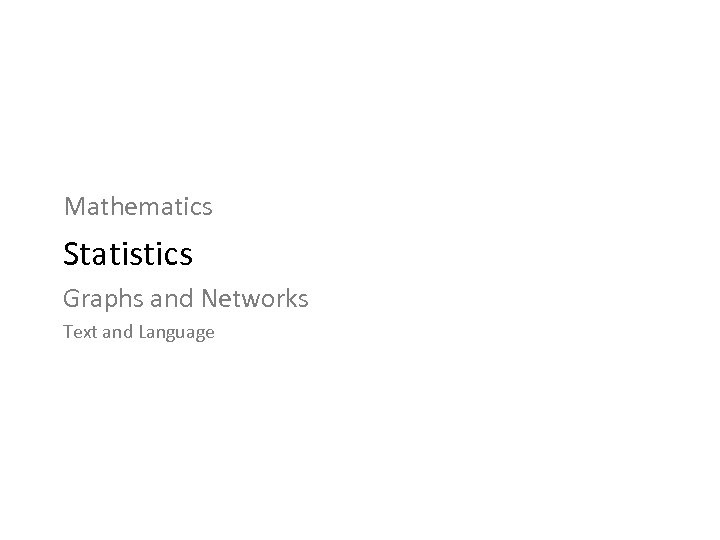Mathematics Statistics Graphs and Networks Text and Language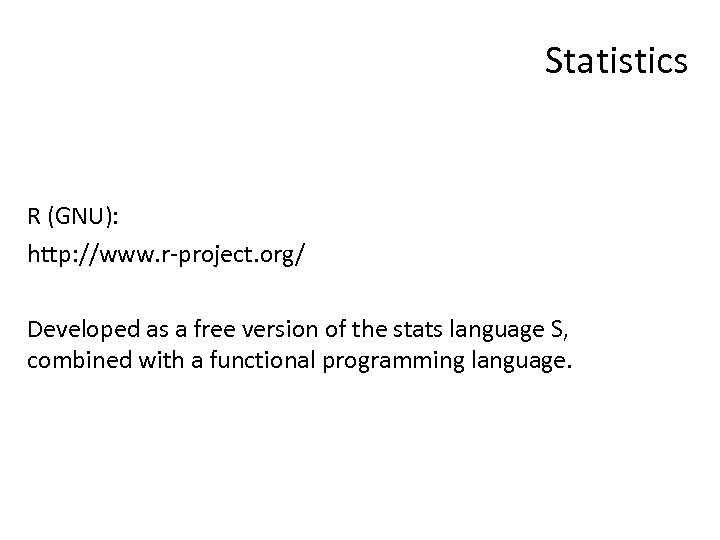Statistics R (GNU): http: //www. r-project. org/ Developed as a free version of the stats language S, combined with a functional programming language.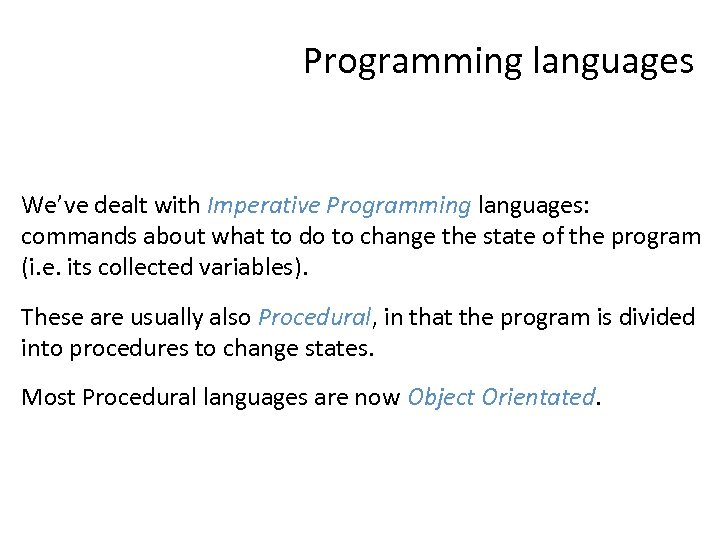Programming languages We’ve dealt with Imperative Programming languages: commands about what to do to change the state of the program (i. e. its collected variables). These are usually also Procedural, in that the program is divided into procedures to change states. Most Procedural languages are now Object Orientated.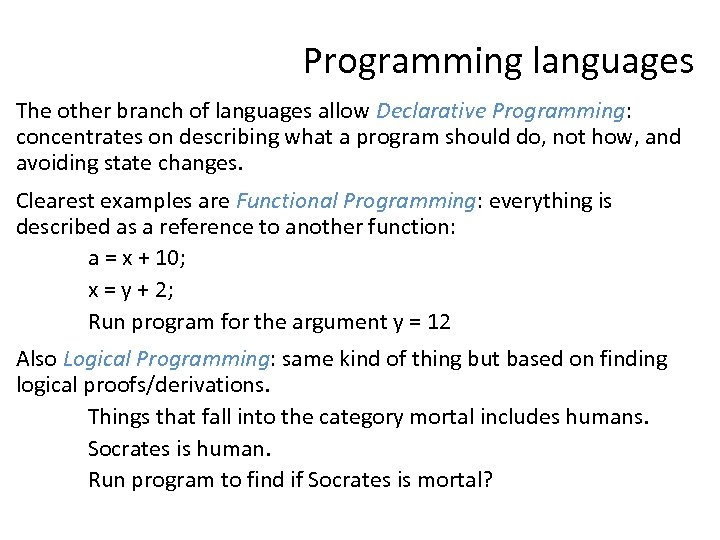Programming languages The other branch of languages allow Declarative Programming: concentrates on describing what a program should do, not how, and avoiding state changes. Clearest examples are Functional Programming: everything is described as a reference to another function: a = x + 10; x = y + 2; Run program for the argument y = 12 Also Logical Programming: same kind of thing but based on finding logical proofs/derivations. Things that fall into the category mortal includes humans. Socrates is human. Run program to find if Socrates is mortal?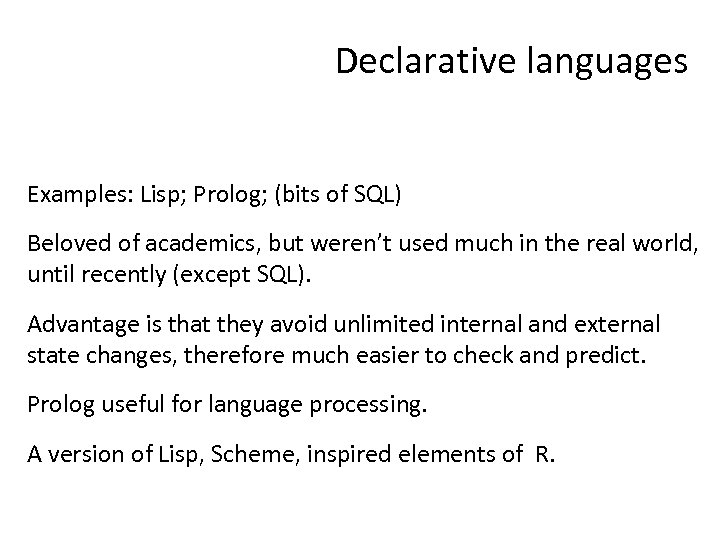Declarative languages Examples: Lisp; Prolog; (bits of SQL) Beloved of academics, but weren’t used much in the real world, until recently (except SQL). Advantage is that they avoid unlimited internal and external state changes, therefore much easier to check and predict. Prolog useful for language processing. A version of Lisp, Scheme, inspired elements of R.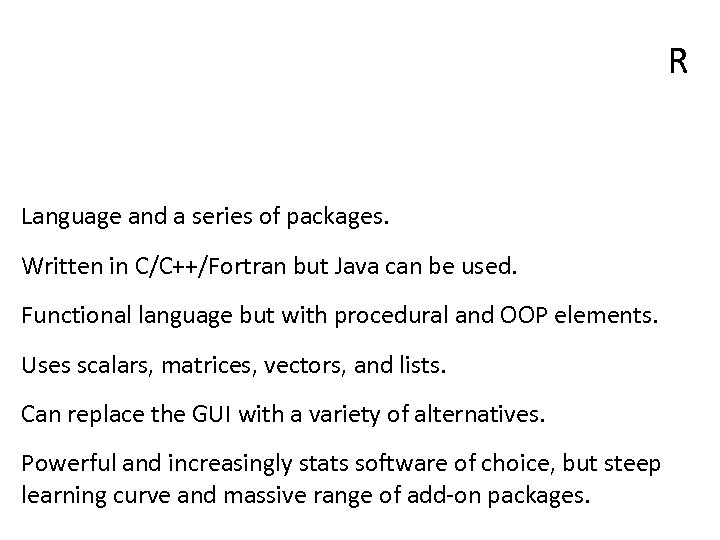R Language and a series of packages. Written in C/C++/Fortran but Java can be used. Functional language but with procedural and OOP elements. Uses scalars, matrices, vectors, and lists. Can replace the GUI with a variety of alternatives. Powerful and increasingly stats software of choice, but steep learning curve and massive range of add-on packages.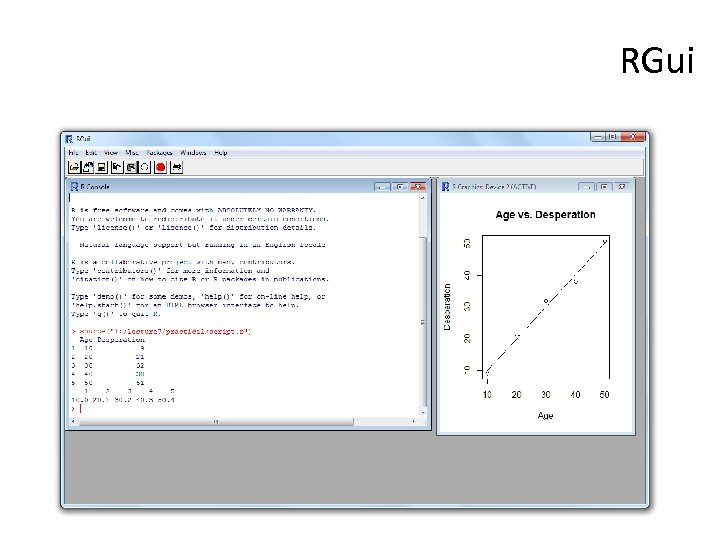RGui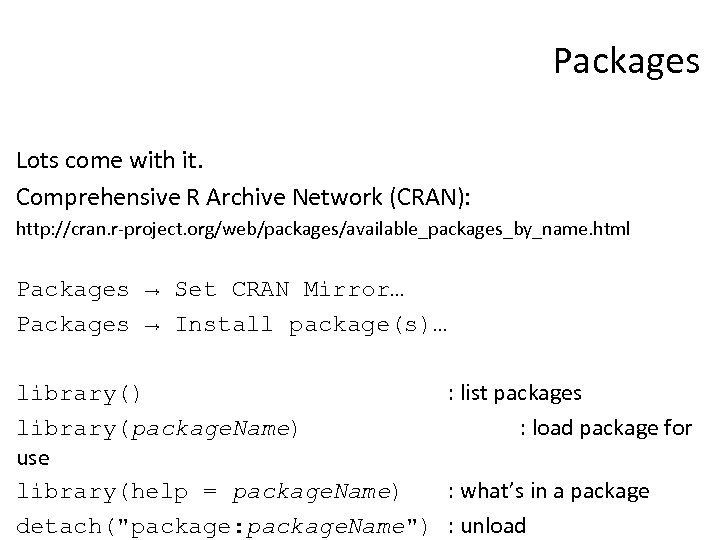Packages Lots come with it. Comprehensive R Archive Network (CRAN): http: //cran. r-project. org/web/packages/available_packages_by_name. html Packages → Set CRAN Mirror… Packages → Install package(s)… library() : list packages library(package. Name) : load package for use library(help = package. Name) : what’s in a package detach("package: package. Name") : unload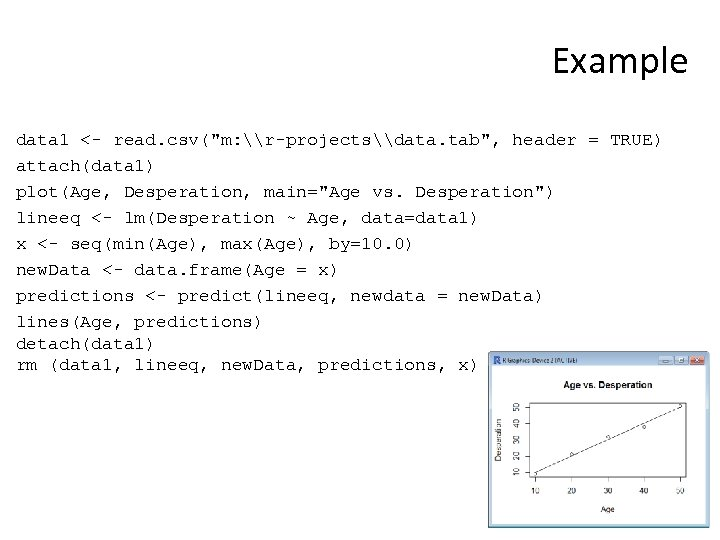Example data 1 <- read. csv("m: \r-projects\data. tab", header = TRUE) attach(data 1) plot(Age, Desperation, main="Age vs. Desperation") lineeq <- lm(Desperation ~ Age, data=data 1) x <- seq(min(Age), max(Age), by=10. 0) new. Data <- data. frame(Age = x) predictions <- predict(lineeq, newdata = new. Data) lines(Age, predictions) detach(data 1) rm (data 1, lineeq, new. Data, predictions, x)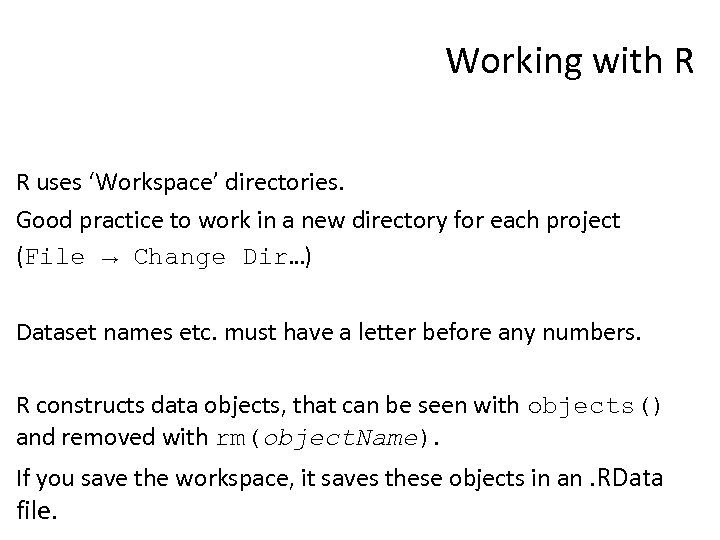Working with R R uses ‘Workspace’ directories. Good practice to work in a new directory for each project (File → Change Dir…) Dataset names etc. must have a letter before any numbers. R constructs data objects, that can be seen with objects() and removed with rm(object. Name). If you save the workspace, it saves these objects in an. RData file.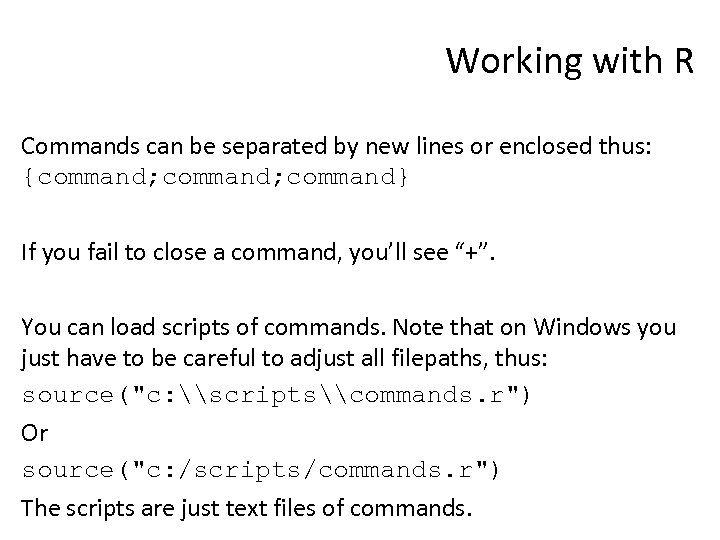Working with R Commands can be separated by new lines or enclosed thus: {command; command} If you fail to close a command, you’ll see “+”. You can load scripts of commands. Note that on Windows you just have to be careful to adjust all filepaths, thus: source("c: \scripts\commands. r") Or source("c: /scripts/commands. r") The scripts are just text files of commands.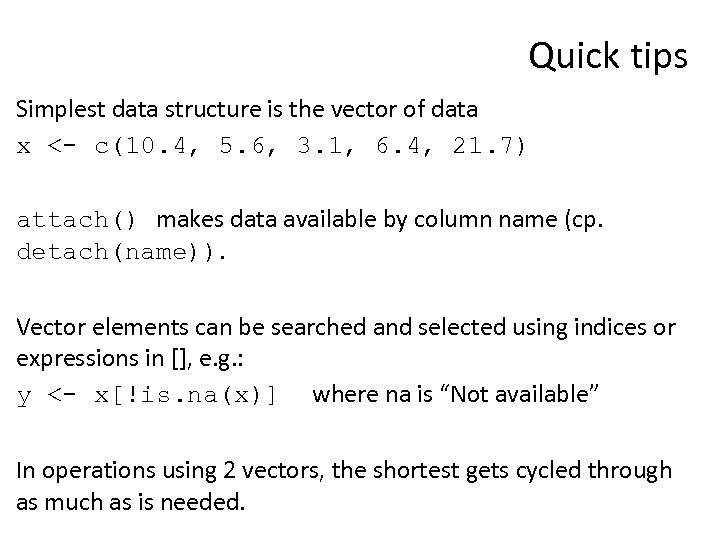Quick tips Simplest data structure is the vector of data x <- c(10. 4, 5. 6, 3. 1, 6. 4, 21. 7) attach() makes data available by column name (cp. detach(name)). Vector elements can be searched and selected using indices or expressions in [], e. g. : y <- x[!is. na(x)] where na is “Not available” In operations using 2 vectors, the shortest gets cycled through as much as is needed.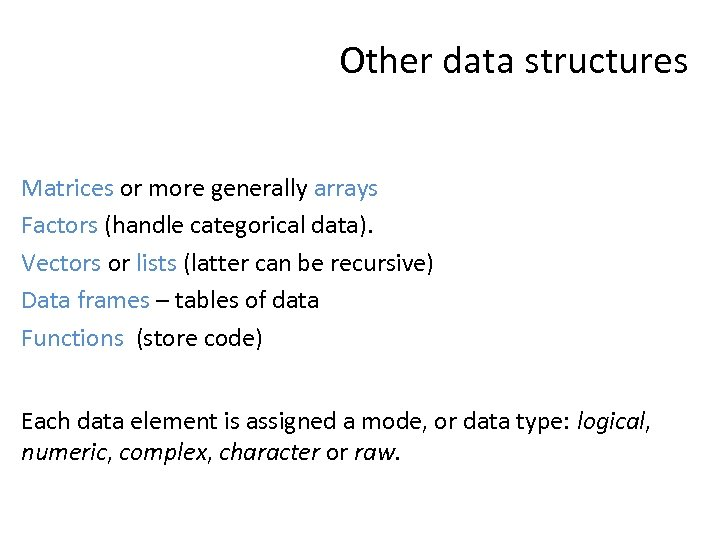Other data structures Matrices or more generally arrays Factors (handle categorical data). Vectors or lists (latter can be recursive) Data frames – tables of data Functions (store code) Each data element is assigned a mode, or data type: logical, numeric, complex, character or raw.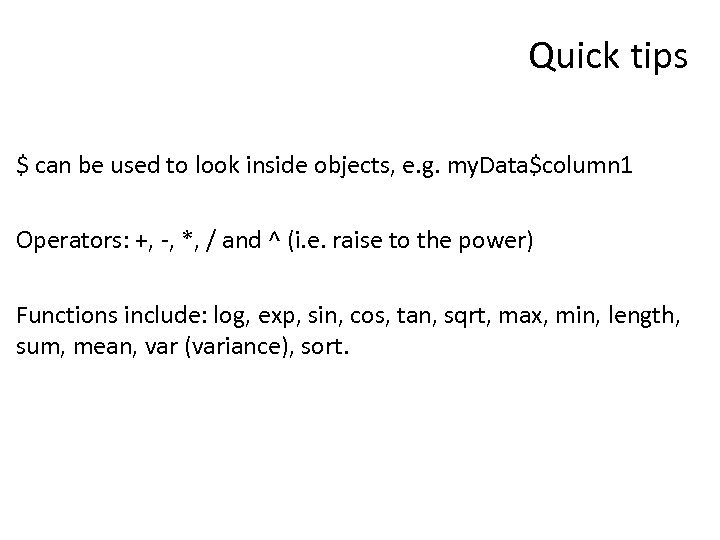Quick tips \$ can be used to look inside objects, e. g. my. Data\$column 1 Operators: +, -, *, / and ^ (i. e. raise to the power) Functions include: log, exp, sin, cos, tan, sqrt, max, min, length, sum, mean, var (variance), sort.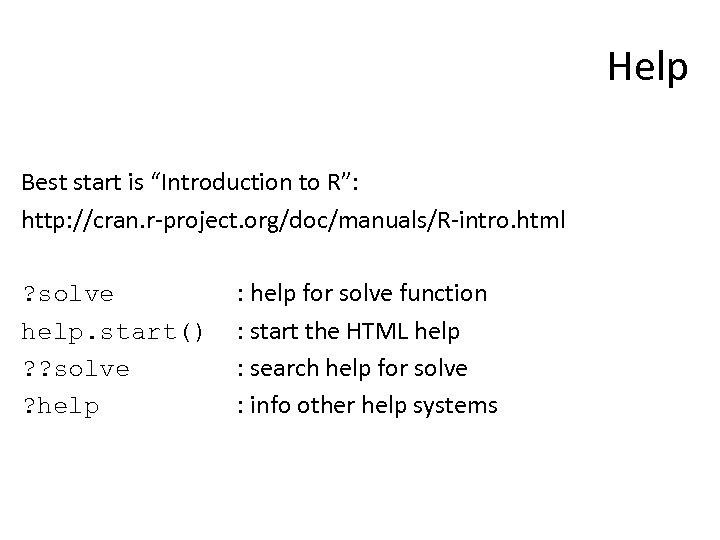Help Best start is “Introduction to R”: http: //cran. r-project. org/doc/manuals/R-intro. html ? solve help. start() ? ? solve ? help : help for solve function : start the HTML help : search help for solve : info other help systems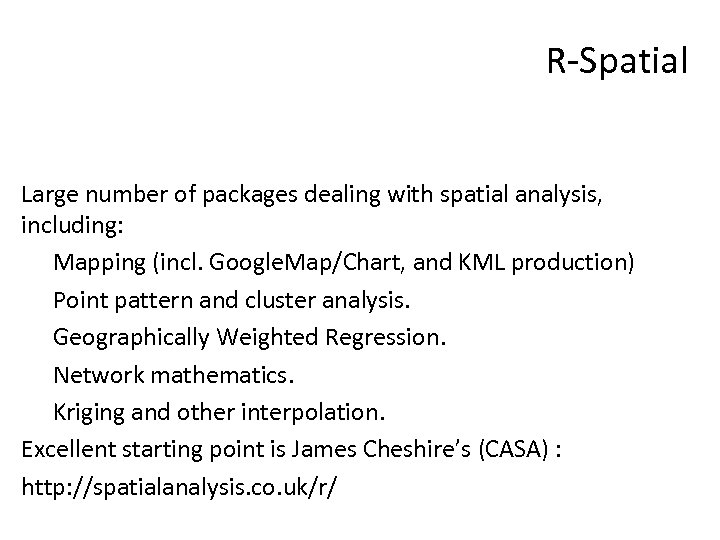R-Spatial Large number of packages dealing with spatial analysis, including: Mapping (incl. Google. Map/Chart, and KML production) Point pattern and cluster analysis. Geographically Weighted Regression. Network mathematics. Kriging and other interpolation. Excellent starting point is James Cheshire’s (CASA) : http: //spatialanalysis. co. uk/r/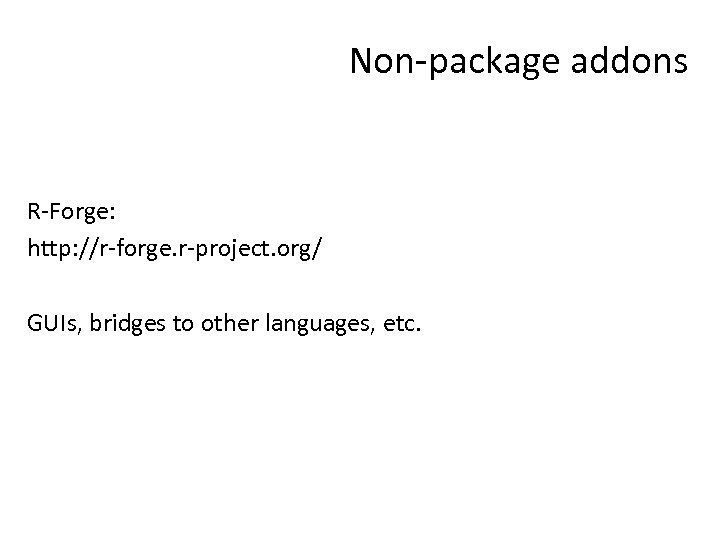Non-package addons R-Forge: http: //r-forge. r-project. org/ GUIs, bridges to other languages, etc.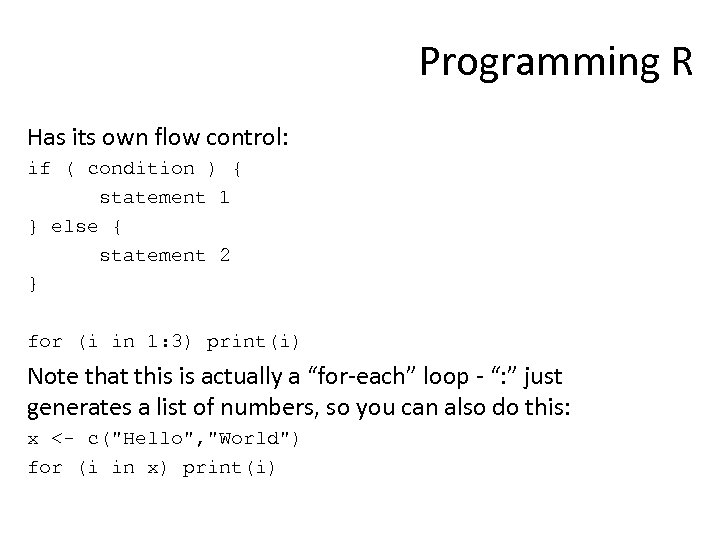Programming R Has its own flow control: if ( condition ) { statement 1 } else { statement 2 } for (i in 1: 3) print(i) Note that this is actually a “for-each” loop - “: ” just generates a list of numbers, so you can also do this: x <- c("Hello", "World") for (i in x) print(i)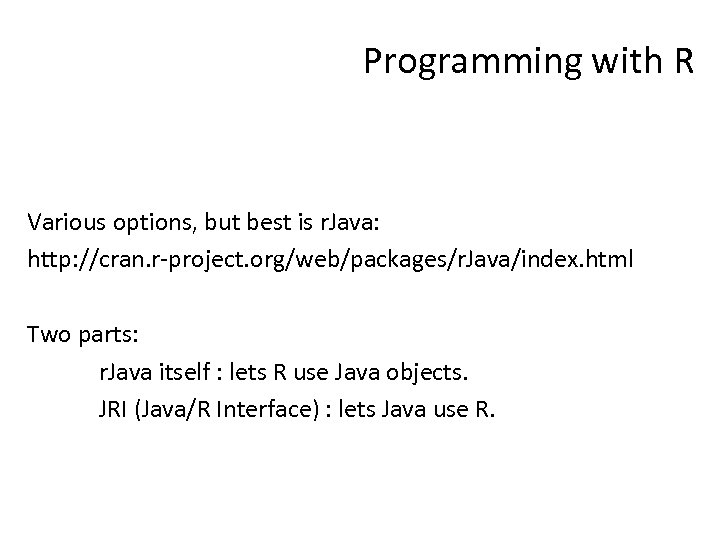Programming with R Various options, but best is r. Java: http: //cran. r-project. org/web/packages/r. Java/index. html Two parts: r. Java itself : lets R use Java objects. JRI (Java/R Interface) : lets Java use R.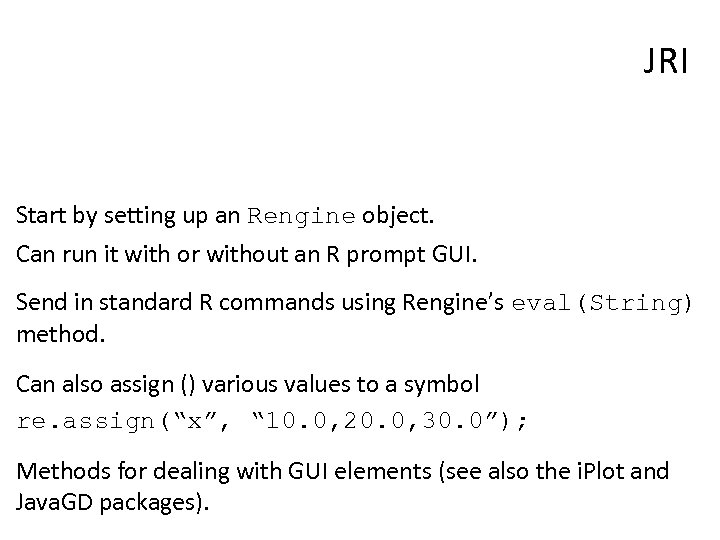JRI Start by setting up an Rengine object. Can run it with or without an R prompt GUI. Send in standard R commands using Rengine’s eval(String) method. Can also assign () various values to a symbol re. assign(“x”, “ 10. 0, 20. 0, 30. 0”); Methods for dealing with GUI elements (see also the i. Plot and Java. GD packages).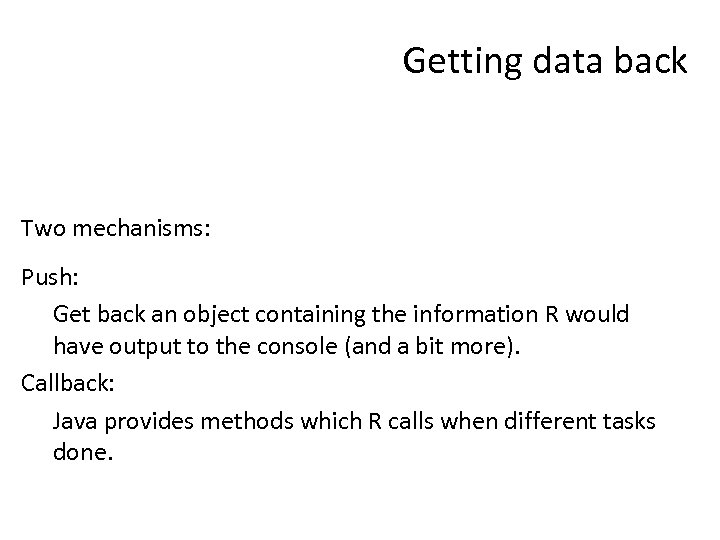Getting data back Two mechanisms: Push: Get back an object containing the information R would have output to the console (and a bit more). Callback: Java provides methods which R calls when different tasks done.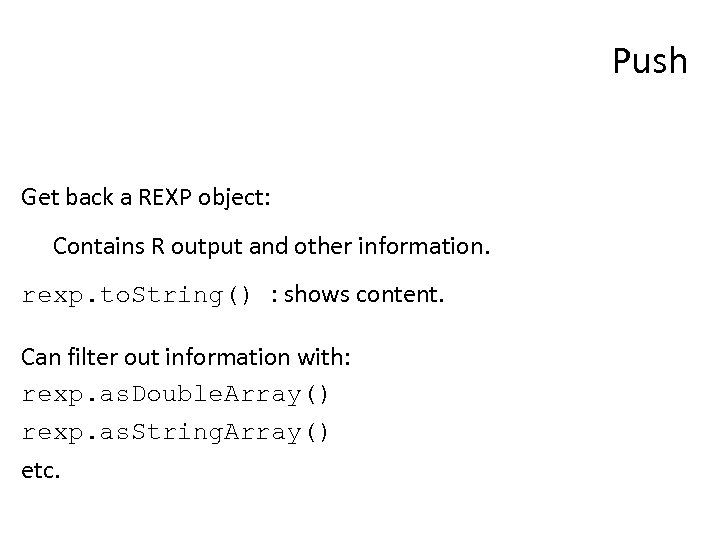Push Get back a REXP object: Contains R output and other information. rexp. to. String() : shows content. Can filter out information with: rexp. as. Double. Array() rexp. as. String. Array() etc.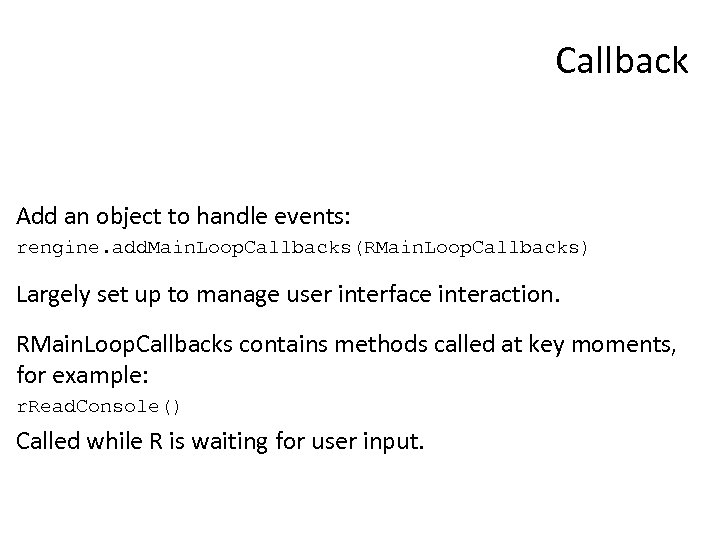Callback Add an object to handle events: rengine. add. Main. Loop. Callbacks(RMain. Loop. Callbacks) Largely set up to manage user interface interaction. RMain. Loop. Callbacks contains methods called at key moments, for example: r. Read. Console() Called while R is waiting for user input.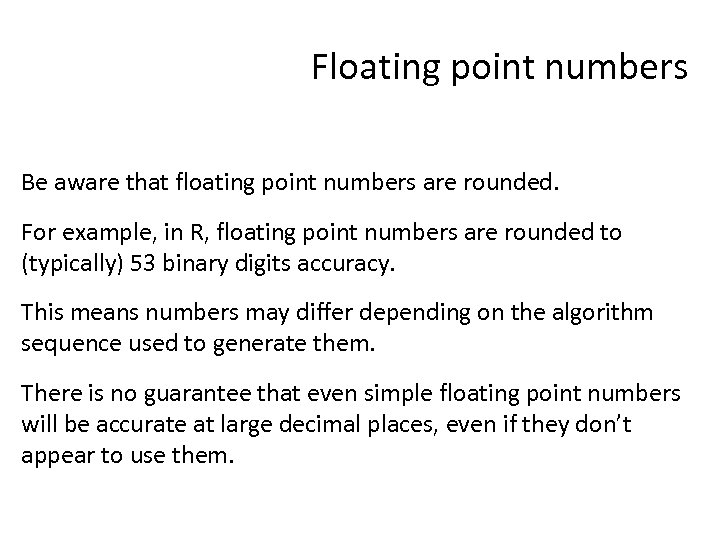Floating point numbers Be aware that floating point numbers are rounded. For example, in R, floating point numbers are rounded to (typically) 53 binary digits accuracy. This means numbers may differ depending on the algorithm sequence used to generate them. There is no guarantee that even simple floating point numbers will be accurate at large decimal places, even if they don’t appear to use them.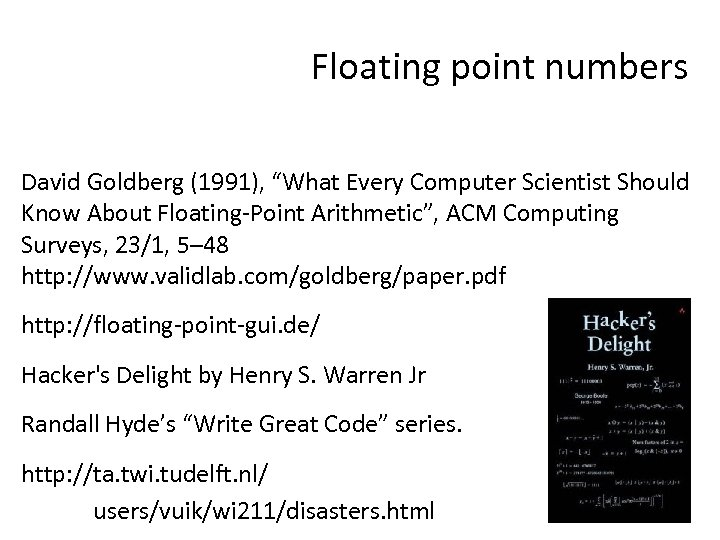Floating point numbers David Goldberg (1991), “What Every Computer Scientist Should Know About Floating-Point Arithmetic”, ACM Computing Surveys, 23/1, 5– 48 http: //www. validlab. com/goldberg/paper. pdf http: //floating-point-gui. de/ Hacker's Delight by Henry S. Warren Jr Randall Hyde’s “Write Great Code” series. http: //ta. twi. tudelft. nl/ users/vuik/wi 211/disasters. html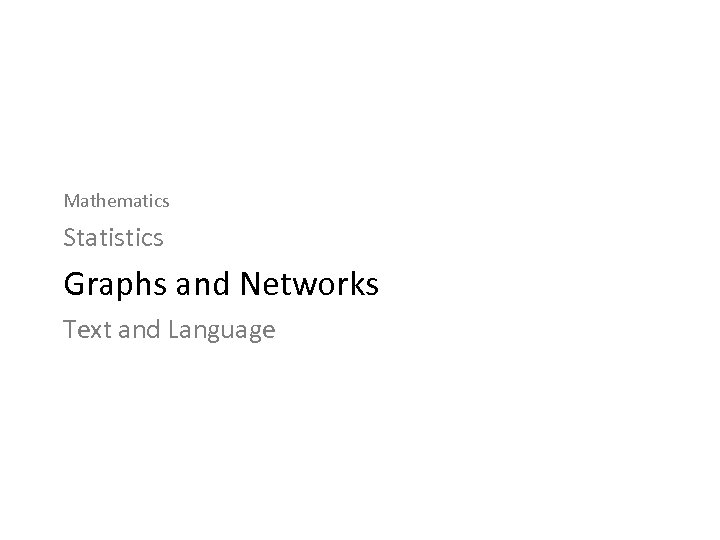Mathematics Statistics Graphs and Networks Text and Language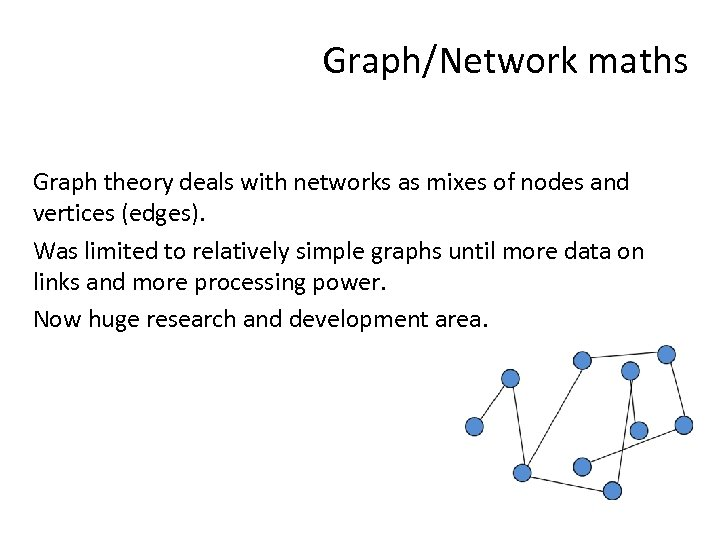Graph/Network maths Graph theory deals with networks as mixes of nodes and vertices (edges). Was limited to relatively simple graphs until more data on links and more processing power. Now huge research and development area.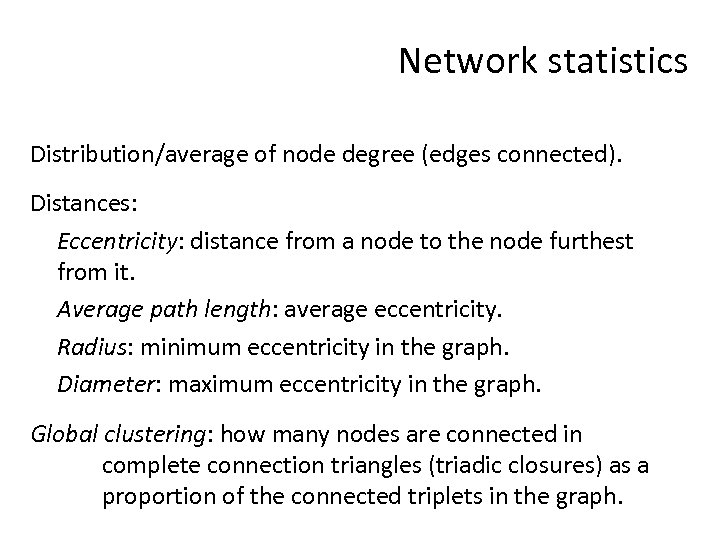Network statistics Distribution/average of node degree (edges connected). Distances: Eccentricity: distance from a node to the node furthest from it. Average path length: average eccentricity. Radius: minimum eccentricity in the graph. Diameter: maximum eccentricity in the graph. Global clustering: how many nodes are connected in complete connection triangles (triadic closures) as a proportion of the connected triplets in the graph.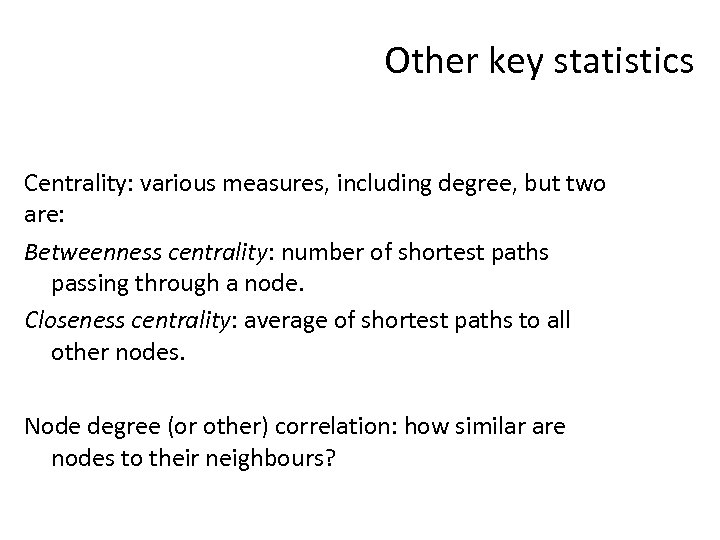Other key statistics Centrality: various measures, including degree, but two are: Betweenness centrality: number of shortest paths passing through a node. Closeness centrality: average of shortest paths to all other nodes. Node degree (or other) correlation: how similar are nodes to their neighbours?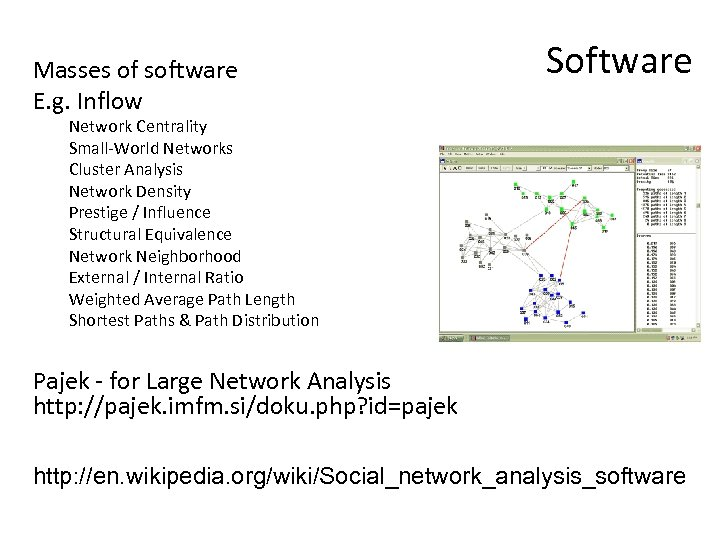Masses of software E. g. Inflow Software Network Centrality Small-World Networks Cluster Analysis Network Density Prestige / Influence Structural Equivalence Network Neighborhood External / Internal Ratio Weighted Average Path Length Shortest Paths & Path Distribution Pajek - for Large Network Analysis http: //pajek. imfm. si/doku. php? id=pajek http: //en. wikipedia. org/wiki/Social_network_analysis_software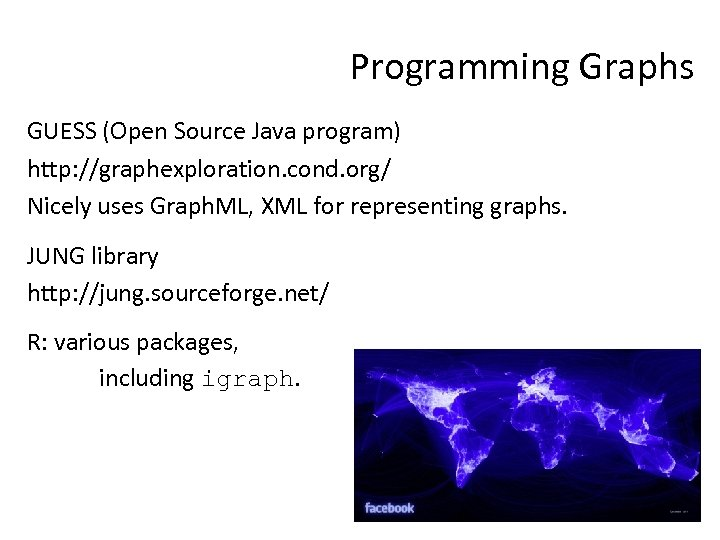Programming Graphs GUESS (Open Source Java program) http: //graphexploration. cond. org/ Nicely uses Graph. ML, XML for representing graphs. JUNG library http: //jung. sourceforge. net/ R: various packages, including igraph.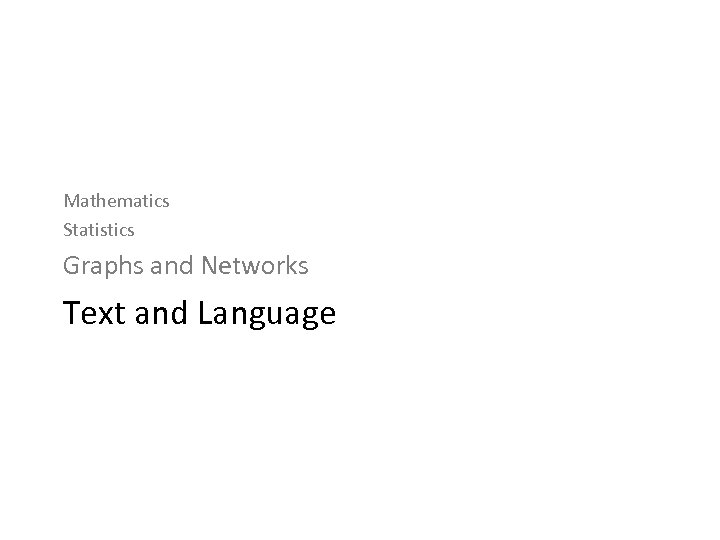Mathematics Statistics Graphs and Networks Text and Language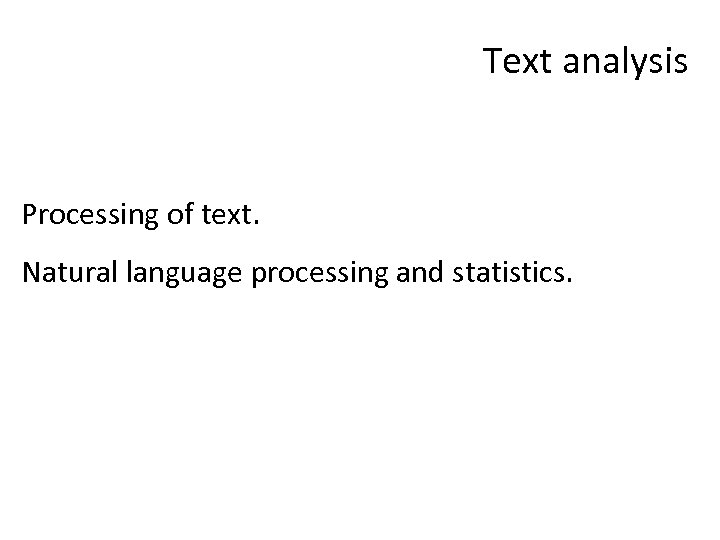Text analysis Processing of text. Natural language processing and statistics.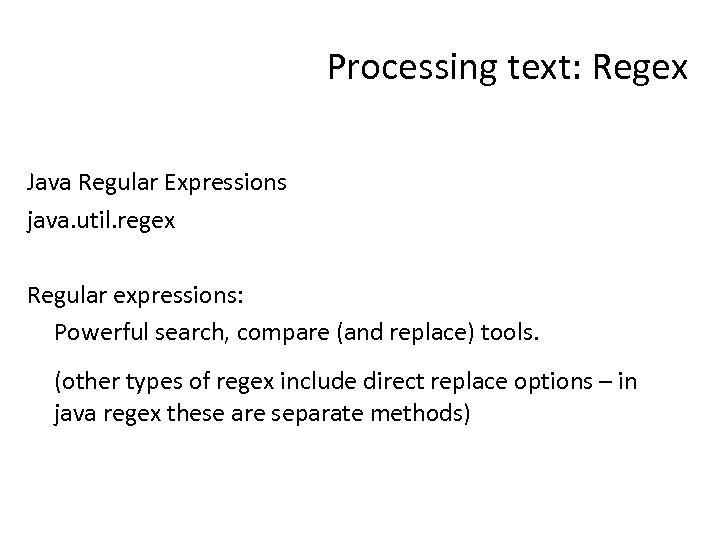Processing text: Regex Java Regular Expressions java. util. regex Regular expressions: Powerful search, compare (and replace) tools. (other types of regex include direct replace options – in java regex these are separate methods)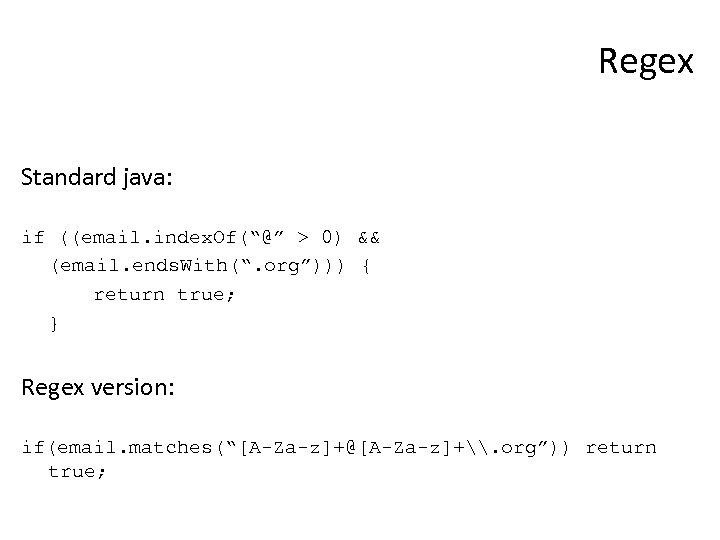Regex Standard java: if ((email. index. Of(“@” > 0) && (email. ends. With(“. org”))) { return true; } Regex version: if(email. matches(“[A-Za-z][email protected][A-Za-z]+\. org”)) return true;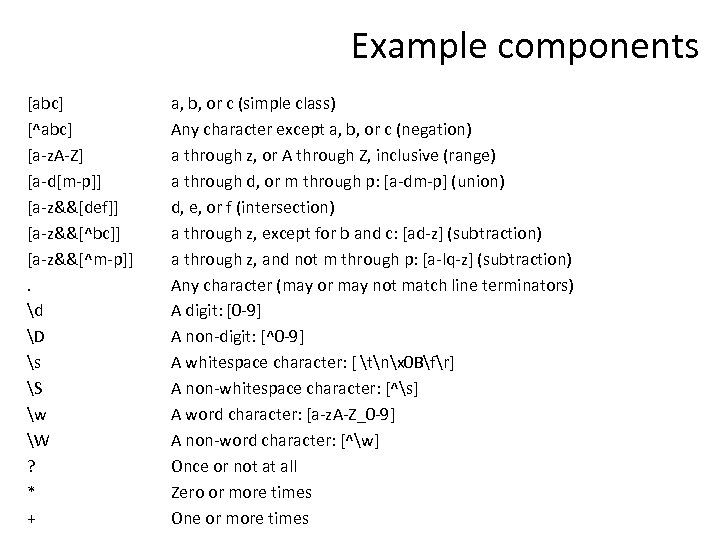Example components [abc] [^abc] [a-z. A-Z] [a-d[m-p]] [a-z&&[def]] [a-z&&[^bc]] [a-z&&[^m-p]]. d D s S w W ? * + a, b, or c (simple class) Any character except a, b, or c (negation) a through z, or A through Z, inclusive (range) a through d, or m through p: [a-dm-p] (union) d, e, or f (intersection) a through z, except for b and c: [ad-z] (subtraction) a through z, and not m through p: [a-lq-z] (subtraction) Any character (may or may not match line terminators) A digit: [0 -9] A non-digit: [^0 -9] A whitespace character: [ tnx 0 Bfr] A non-whitespace character: [^s] A word character: [a-z. A-Z_0 -9] A non-word character: [^w] Once or not at all Zero or more times One or more times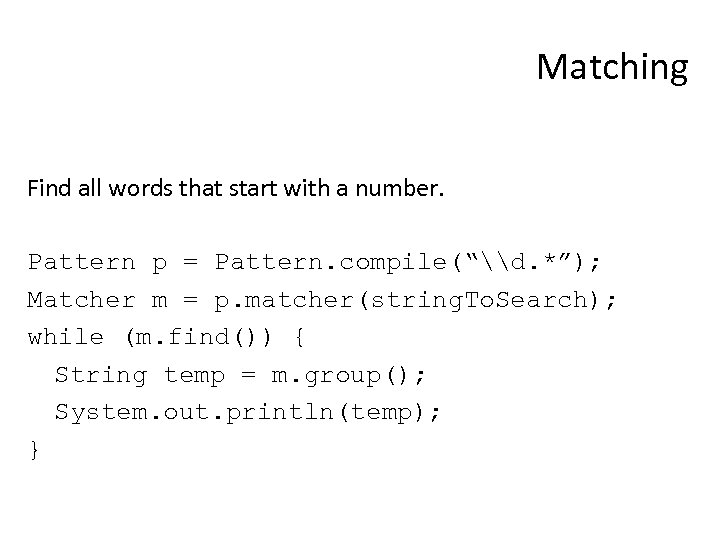Matching Find all words that start with a number. Pattern p = Pattern. compile(“\d. *”); Matcher m = p. matcher(string. To. Search); while (m. find()) { String temp = m. group(); System. out. println(temp); }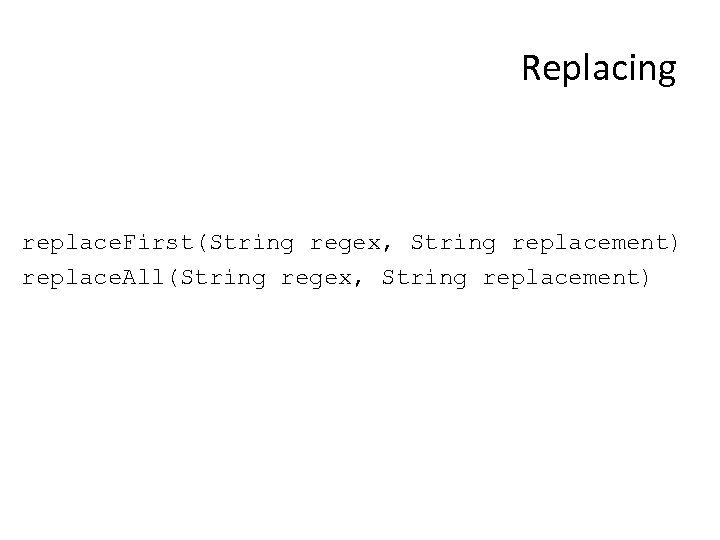Replacing replace. First(String regex, String replacement) replace. All(String regex, String replacement)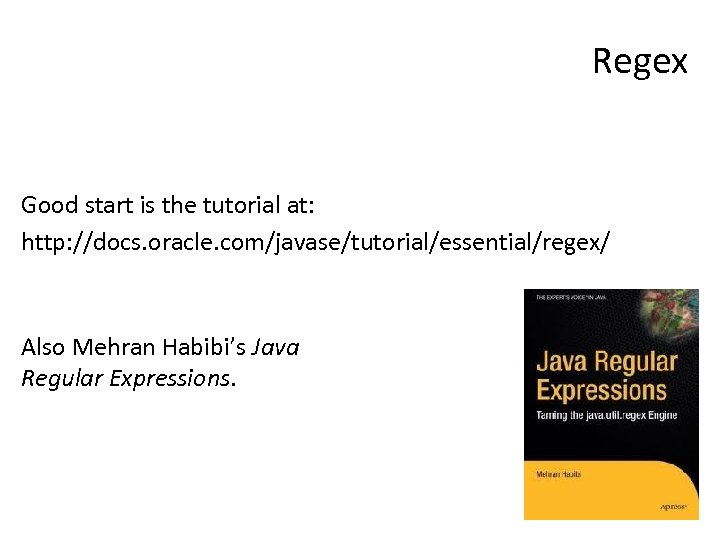Regex Good start is the tutorial at: http: //docs. oracle. com/javase/tutorial/essential/regex/ Also Mehran Habibi’s Java Regular Expressions.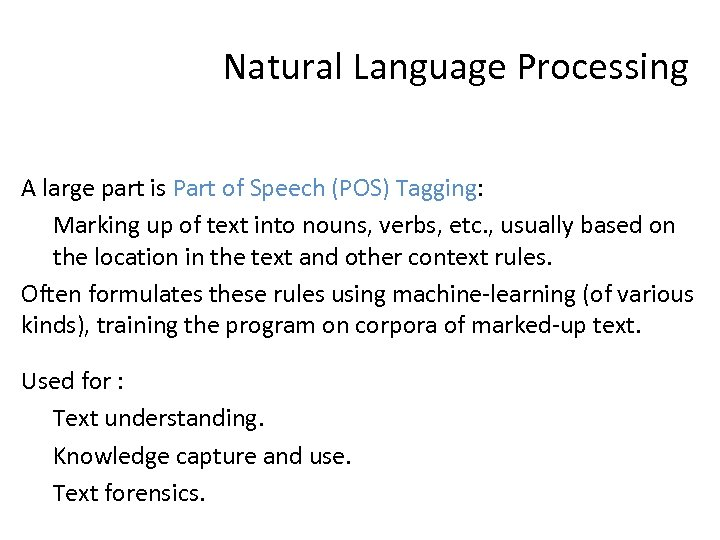Natural Language Processing A large part is Part of Speech (POS) Tagging: Marking up of text into nouns, verbs, etc. , usually based on the location in the text and other context rules. Often formulates these rules using machine-learning (of various kinds), training the program on corpora of marked-up text. Used for : Text understanding. Knowledge capture and use. Text forensics.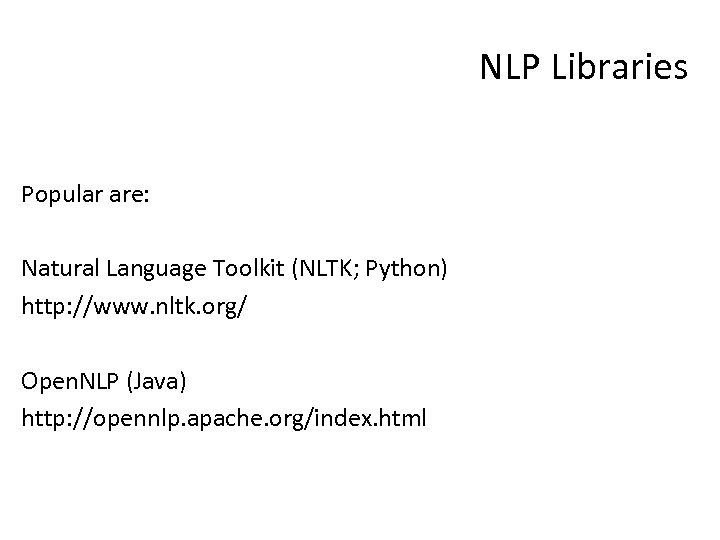NLP Libraries Popular are: Natural Language Toolkit (NLTK; Python) http: //www. nltk. org/ Open. NLP (Java) http: //opennlp. apache. org/index. html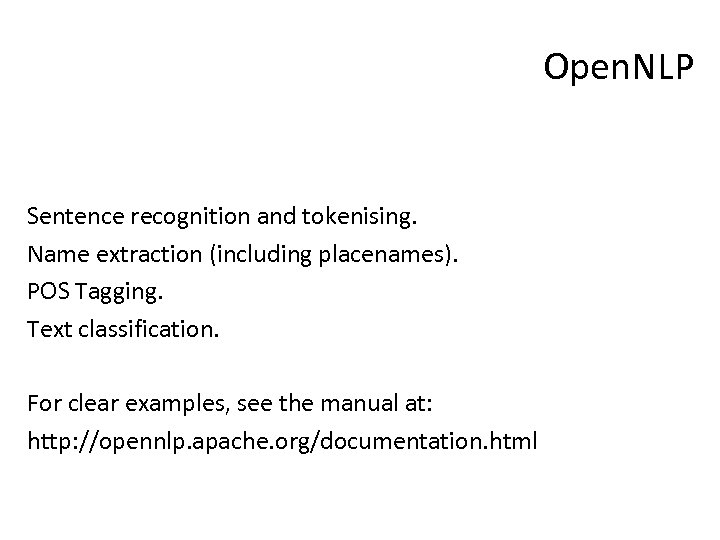Open. NLP Sentence recognition and tokenising. Name extraction (including placenames). POS Tagging. Text classification. For clear examples, see the manual at: http: //opennlp. apache. org/documentation. html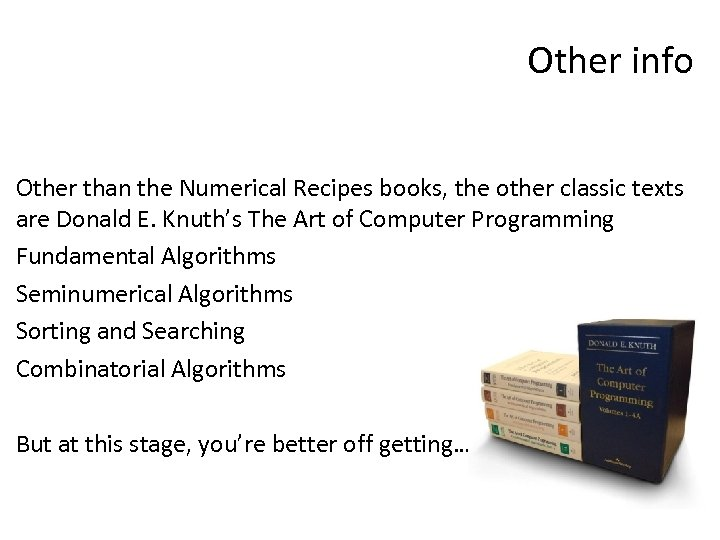Other info Other than the Numerical Recipes books, the other classic texts are Donald E. Knuth’s The Art of Computer Programming Fundamental Algorithms Seminumerical Algorithms Sorting and Searching Combinatorial Algorithms But at this stage, you’re better off getting…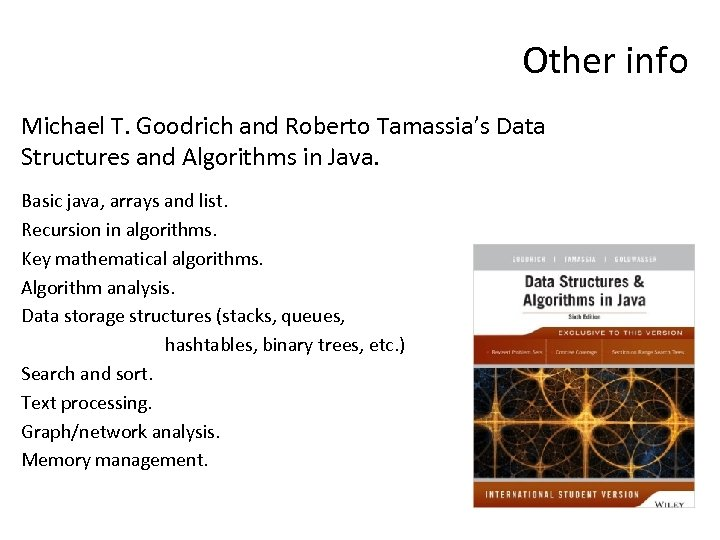Other info Michael T. Goodrich and Roberto Tamassia’s Data Structures and Algorithms in Java. Basic java, arrays and list. Recursion in algorithms. Key mathematical algorithms. Algorithm analysis. Data storage structures (stacks, queues, hashtables, binary trees, etc. ) Search and sort. Text processing. Graph/network analysis. Memory management.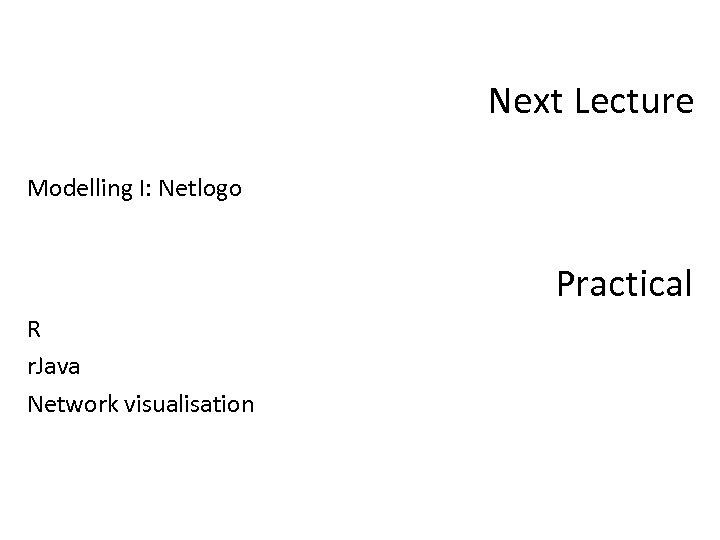Next Lecture Modelling I: Netlogo Practical R r. Java Network visualisation